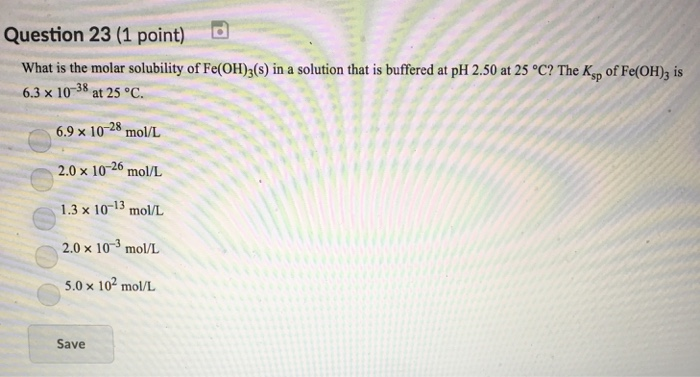# Question & Answer: What is the molar solubility of Fe(OH)3 in a solution thatvis buffered at pH 2.50…..What is the molar solubility of Fe(OH)3 in a solution thatvis buffered at pH 2.50

pH = 2.50

Don't use plagiarized sources. Get Your Custom Essay on
Question & Answer: What is the molar solubility of Fe(OH)3 in a solution thatvis buffered at pH 2.50…..
GET AN ESSAY WRITTEN FOR YOU FROM AS LOW AS \$13/PAGE

pOH = 14-pH = 14-2.50 = 11.5

pOH = -log[OH]

[OH] = antilog(-pOH)

= antilog(-11.5)

[OH] = 3.16*10-12M

Let the solubility be S moles/L

Fe(OH)3 <—> Fe3+ + 3OH

Ksp = [Fe3+][OH]3

6.3*10-38 = S*(3.16*10-12)3

S = 6.3*10-38 / 31.55*10-36

S = 1.99*10-3mol/L = 2.0*10-3mol/L

Option D